# Trigonometric Functions

These Trigonometric Functions blocks perform trigonometry operations on an input number and return an output number.

Block Icon Description
Arc Cosine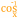Returns the arccosine, or inverse cosine, of a number, in degrees
Arc Sine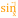Returns the arcsine, or inverse sine, of a number, in degrees
Arc Tangent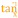Returns the arctangent, or inverse tangent, of a number, in degrees
Cosine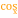Returns the cosine of the given angle
Cotangent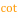Returns the cotangent of an angle, in degrees
Degree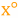Converts radians to degrees
Radian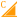Converts degrees to radians
Sine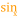Returns the sine of the given angle
Tangent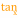Returns the tangent of the given angle

2018/10/03 00:17